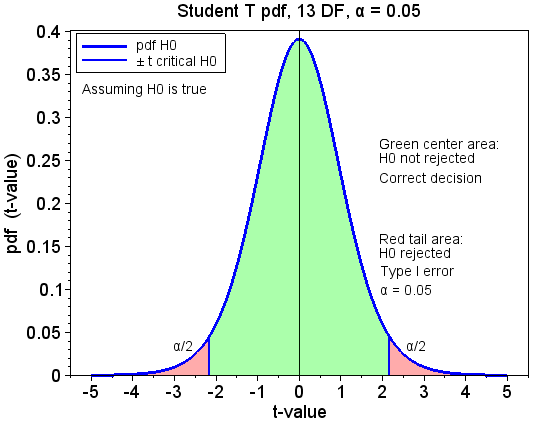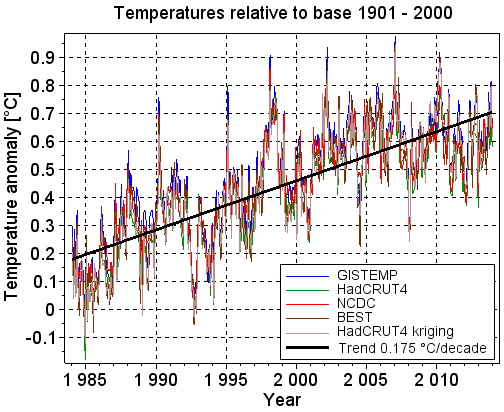## søndag 22. juni 2014

### Piecewise linear regression applied to temperature trends

This is the fifth blog post in a series of five that analyse trends in the global surface temperatures. The posts put emphasis on the mathematics and the statistics used in the analyses. The posts are numbered 1 to 5. They should be read consecutively.

Post 1    Linear regression analysis
Post 2    Hypothesis testing of temperature trends
Post 3    Confidence intervals around temperature trend lines
Post 4    Statistical power of temperature trends
Post 5    Piecewise linear regression applied to temperature trends

The posts are gathered in this pdf document.

Start of post 5 Piecewise linear regression applied to temperature trends

The temperature trend line from December 2000 to December 2013 is flat, while the one from January 1984 to November 2000 increases with 0.22°C/decade, as shown with the two blue lines in Figure 5.1.Figure 5.1: Monthly temperatures in the last 30 years with trend lines

This leads many contrarians to argue that the increasing temperature trend before the turn of the millennium is followed by a flat trend, and they often illustrate their claim with the red schematic line in Figure 5.1. The red line is, however, not based on calculations, and it does not match the monthly temperatures that it claims to represent. The two blue lines are calculated with linear regression analysis, and they represent the temperatures in their segments when the segments are evaluated isolated from each other. But the trend lines are not continuous at the breakpoint between November and December 2000, and they therefore do not represent the trend for the whole time period in Figure 5.1.

We may calculate a piecewise linear trend line that is continuous at the breakpoint. This new trend line is a “best fit” to the temperatures in the whole time period, just as the two blue lines are the best fits for their time periods. The new trend line has an increasing trend also after the turn of the millennium, as the green line in Figure 5.1 shows. It is calculated with piecewise linear regression analysis.

## fredag 20. juni 2014

### Statistical power of temperature trends

This is the fourth blog post in a series of five that analyse trends in the global surface temperatures. The posts put emphasis on the mathematics and the statistics used in the analyses. The posts are numbered 1 to 5. They should be read consecutively.

Post 1    Linear regression analysis
Post 2    Hypothesis testing of temperature trends
Post 3    Confidence intervals around temperature trend lines
Post 4    Statistical power of temperature trends
Post 5    Piecewise linear regression applied to temperature trends

The posts are gathered in this pdf document.

Start of post 4 Statistical power of temperature trends

β (beta) is the probability of not rejecting the null hypothesis H0 when it is false. This is a type II error. Statistical power is the probability of rejecting a false null hypothesis. It is 1 minus β.

We assume that the null hypothesis is false and that the alternative hypothesis H1 is true, i.e. that there is a true long term temperature trend different from zero. But we do not know the true trend, only that it is different from zero. The t-value is the slope of the trend divided with its 1-σ uncertainty. We need the t-value of a trend under the alternative hypothesis in order to calculate β and statistical power. In lack of better information we may assume that the trend calculated based on a set of temperature measurements (later called a dataset) is the true trend, and therefore use the t-value of that trend as the t-value under the alternative hypothesis. With this approach we calculate the post-hoc (retrospective) statistical power. Another approach is to estimate the trend and its noise based on available knowledge independent of a specific dataset being analyzed, and thereafter use this for the trend under the alternative hypothesis. With this approach we calculate the a-priori (prospective) statistical power.

## onsdag 18. juni 2014

### Confidence intervals around temperature trend lines

This is the third blog post in a series of five that analyse trends in the global surface temperatures. The posts put emphasis on the mathematics and the statistics used in the analyses. The posts are numbered 1 to 5. They should be read consecutively.

Post 1    Linear regression analysis
Post 2    Hypothesis testing of temperature trends
Post 3    Confidence intervals around temperature trend lines
Post 4    Statistical power of temperature trends
Post 5    Piecewise linear regression applied to temperature trends

The posts are gathered in this pdf document.

Start of post 3, Confidence intervals around temperature trend lines:

Figure 3.1 shows the monthly temperatures in the last 30 years as blue dots. The solid red line shows the temperature trend in these 30 years.Figure 3.1: Monthly temperatures from January 1984 to December 2013 with trend line
The red line is a “best fit” to the blue dots. The slope and the intersection with the vertical Y axis are estimated with linear regression analysis. The slope is defined with its value and its uncertainty, both with units °C/year. The uncertainty is decided by both the length of the interval which the trend is calculated over and by the noise on the temperatures. Long intervals give low uncertainty, and much noise gives high uncertainty. The uncertainty is usually specified with its 1-sigma value σ. See more details in post 1.

The 95% confidence interval around an estimated value has a 95% likelihood of covering the true value. The upper endpoint of the confidence interval has a 97.5% likelihood of exceeding the true value, and the lower endpoint has a 97.5% likelihood of being less than it.

The red regression line in Figure 3.1 may be regarded as a model. It may be used in two different ways. One way is to estimate the most likely temperature at a given time. The red dotted lines show the 95% confidence interval around this estimation. Another way is to predict a measurement at a given time. The blue dotted lines show the 95% confidence interval around this prediction. It is wider than the confidence interval for the estimate because it also includes the uncertainty of the measurement that is being predicted.

## tirsdag 17. juni 2014

### Hypothesis testing of temperature trends

This is the second blog post in a series of five that analyse trends in the global surface temperatures. The posts put emphasis on the mathematics and the statistics used in the analyses. The posts are numbered 1 to 5. They should be read consecutively.

Post 1    Linear regression analysis
Post 2    Hypothesis testing of temperature trends
Post 3    Confidence intervals of temperature trends
Post 4    Statistical power of temperature trends
Post 5    Piecewise linear regression applied to temperature trends

The posts are gathered in this pdf document.

Start of post 2, Hypothesis testing of temperature trends:

The decision of whether a calculated temperature trend is statistically significant or not is based on hypothesis testing. The null hypothesis H0 is that the underlying long term trend is zero and that a calculated trend different from zero is caused by random noise on the measurements. The alternative hypothesis H1 is that the underlying long term trend is different from zero.

The t-value of the trend is its estimated slope [°C/year] divided with its 1-σ uncertainty [°C/year]. It is a dimensionless number. The t-value follows a Student's t-distribution when the noise on the temperature measurements is random. The probability density function (pdf) of the t-distribution is symmetrical and bell-shaped, as shown in Figure 2.1. The degrees of freedom of the t-distribution is the number of independent measurements minus two.

The absolute value of the t-value is a measure of the probability that the slope is different from zero. A t-value less than 1 tells that the uncertainty of the calculated slope is greater than the slope itself; then the true slope may very well be zero. If, however, the t-value is much greater than 1, the true slope is probably different from zero.

When we calculate a temperature trend different from zero, we do not know if it is caused by random noise on the measurements or by a long term trend different from zero. The calculated trend is statistically significant at the α significance level if the probability to calculate such an extreme trend is less than α, given that the null hypothesis is true. The term 'Such an extreme trend' means a trend that is as big as or even bigger than the calculated trend, positive or negative. This is illustrated in Figure 2.1, which shows the pdf of the t-value under the null hypothesis.Figure 2.1: The Student's t-distribution with illustration of the significance level α equal to 0.05.The plot assumes that the null hypothesis H0 is true.

## mandag 16. juni 2014

### Linear regression analysis

This is the first blog post in a series of five that analyse trends in the global surface temperatures. The posts put emphasis on the mathematics and the statistics used in the analyses. The posts are numbered 1 to 5. They should be read consecutively.

Post 1    Linear regression analysis
Post 2    Hypothesis testing of temperature trends
Post 3    Confidence intervals of temperature trends
Post 4    Statistical power of temperature trends
Post 5    Piecewise linear regression applied to temperature trends

The posts are gathered in this pdf document.

Start of post 1 Linear regression analysis

The colored lines in Figure 1.1 show the monthly temperature anomalies from January 1984 to December 2013 for five different temperature series. A temperature anomaly is the difference between the real temperature and the average temperature. A base period is a time interval in which the average temperature is calculated. For brevity we often write temperature instead of temperature anomaly. The five series contain the global land and ocean surface temperature anomalies.Figure 1.1: Temperatures in the last 30 years for five different temperature series. The trend line is calculated based on the average of the temperature series.